## Completion Of Incomplete Pattern

#### Completion Of Incomplete Pattern

Direction: In each of the following questions, find out which of the answer figures (a), (b), (c) and (d) completes the figure?

1. Following Question has four alternatives, among which one completes the figures.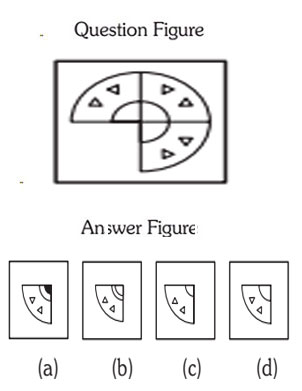##### Correct Option: D

1. Select a suitable figure from the four alternatives that would complete the figure.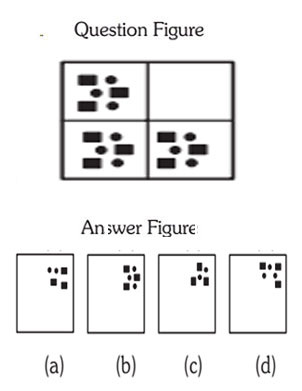##### Correct Option: B

1. Following Question has four alternatives, among which one completes the figures.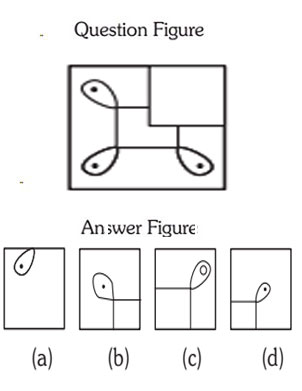##### Correct Option: D

Direction: In the following questions, there are four answer figures which can be formed from the cut out pieces given in question figure.

1. Select a suitable figure from the four alternatives that would complete the figure.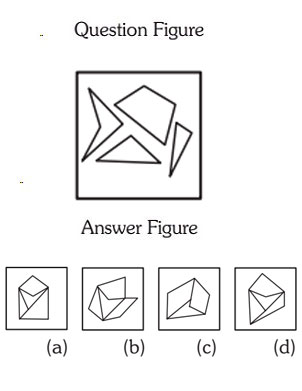1. Option (d) there are two triangles and two quadrilaterals and figure (d) only satisfies it and resembles the figure.

##### Correct Option: D

Option (d) there are two triangles and two quadrilaterals and figure (d) only satisfies it and resembles the figure.

1. Following Question has four alternatives, among which one completes the figures.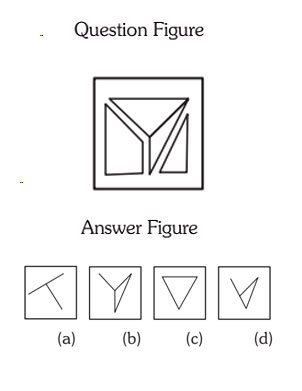1. Option (b) the figure in option (b) is one of the part of the question figure.

##### Correct Option: B

Option (b) the figure in option (b) is one of the part of the question figure.